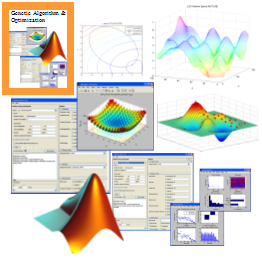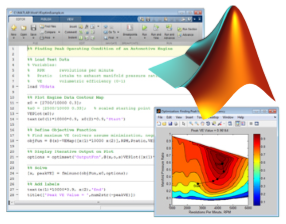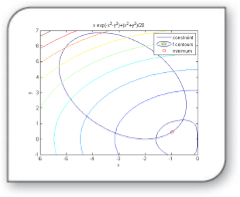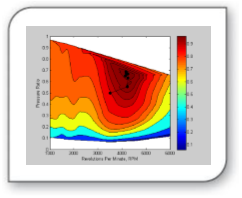Training on Searching for Optimal Solution with Traditional & AI Optimization Techniques Solutions 4U Training Center @ Puchong11 & 12 JAN 2021
" Defining and solving optimization programs
and monitoring solution progress..."
 Optimization can be broadly defined as the process of selecting a set of best parameters from some available alternatives subjecting to stated constraints and meeting specific objectives. Generally, the optimization process involves maximizing or minimizing a real mathematic function by systematically choosing input values from within an allowed set and computing the value of the function. Due to its nature, optimization has found broad usage in engineering and science. For example in automotive industry, optimization can be used to determine the best drive-train settings to minimize emissions. On the other hand in financial sector, optimization can be used to determine the best investment options to minimize risks. In general, optimization process can be implemented and solved by using both traditional methods and artificial-intelligence (AI) techniques such as genetic algorithm (GA).This course is intended as a practical introduction to solving optimization problems using traditional optimization techniques and genetic algorithm. As such, there will be a series of hands-on exercises which are generally aimed to help translate the problems into practical solving using MATLAB. What you will learn Identify the problem and formulate an objective function to solve it Understand various optimization solvers and choose appropriate optimization solver to solve the problem Understand the basic concepts of genetic algorithm and its implementation for solving optimization problem

Course methodology

The course begins with an overview of optimization fundamentals, guidance on writing objective functions, expressing constraints, choosing solvers to basic concepts of genetic algorithm. The course will be well-supported by MATLAB examples.

Course structure

Day 1 – Traditional Optimization Techniques

• Introduction
• Concepts of optimization
• The optimization process
• Choice of design variables
• Formulation of objective function
• Limits and Bounds of design variables
• Formulation of constraints (linear and nonlinear)
• Using Optimization Tool
• Minimizing a simple mathematical function
• Introduction to solvers
• Linear programming
• Binary integer programming
• Iterative-based method
• Least-squares method
• Tips on choosing the solvers
• Case studies:
• Product design: finding the right parameters
• Communication – finding the optimal positions

Day 2 – Genetic Algorithm

• Introduction
• Genetic representation of numbers
• Binary representation
• Floating-point representation
• Processes of genetic computation
• Creation of initial population
• Fitness scoring
• Fitness scaling
• Selection of parent
• Genetic operators (elite, crossover, mutation)
• Using Optimization Tool
• Minimizing a complex mathematical function
• Case studies:
• Using GA for:
• Unconstraint optimization problem
• Linearly constraint optimization problem
• Nonlinear constraint optimization problemWho should attend

Engineers and scientists at all levels who wish to learn about solving optimization problems with MATLAB. No background in optimization theory is, however, assumed. The detailed course material and many source code listings will be invaluable for both learning and reference.

Prerequisites
A basic knowledge of linear algebra and familiarity with MATLAB working environment.

Note: Due to the nature of the course and the learning expectations, the availability seats are limited. You need to register early to obtain confirmation of your space.